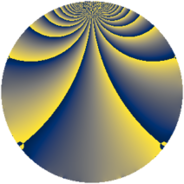# Properties

 Label 3969.1.tLevel $3969$ Weight $1$ Character orbit 3969.t Rep. character $\chi_{3969}(2971,\cdot)$ Character field $\Q(\zeta_{6})$ Dimension $12$ Newform subspaces $5$ Sturm bound $504$ Trace bound $13$

# Related objects

## Defining parameters

 Level: $$N$$ $$=$$ $$3969 = 3^{4} \cdot 7^{2}$$ Weight: $$k$$ $$=$$ $$1$$ Character orbit: $$[\chi]$$ $$=$$ 3969.t (of order $$6$$ and degree $$2$$) Character conductor: $$\operatorname{cond}(\chi)$$ $$=$$ $$63$$ Character field: $$\Q(\zeta_{6})$$ Newform subspaces: $$5$$ Sturm bound: $$504$$ Trace bound: $$13$$

## Dimensions

The following table gives the dimensions of various subspaces of $$M_{1}(3969, [\chi])$$.

Total New Old
Modular forms 112 20 92
Cusp forms 16 12 4
Eisenstein series 96 8 88

The following table gives the dimensions of subspaces with specified projective image type.

$$D_n$$ $$A_4$$ $$S_4$$ $$A_5$$
Dimension 12 0 0 0

## Trace form

 $$12q + 4q^{4} + O(q^{10})$$ $$12q + 4q^{4} - 3q^{13} + 4q^{16} + 4q^{22} - 6q^{25} + q^{37} + q^{43} + 4q^{46} + 3q^{52} + 4q^{58} - 4q^{64} - 6q^{67} - 6q^{79} + 8q^{88} - 3q^{97} + O(q^{100})$$

## Decomposition of $$S_{1}^{\mathrm{new}}(3969, [\chi])$$ into newform subspaces

Label Dim. $$A$$ Field Image CM RM Traces $q$-expansion
$$a_2$$ $$a_3$$ $$a_5$$ $$a_7$$
3969.1.t.a $$2$$ $$1.981$$ $$\Q(\sqrt{-3})$$ $$D_{3}$$ $$\Q(\sqrt{-7})$$ None $$-2$$ $$0$$ $$0$$ $$0$$ $$q-q^{2}+q^{8}-\zeta_{6}^{2}q^{11}-q^{16}+\zeta_{6}^{2}q^{22}+\cdots$$
3969.1.t.b $$2$$ $$1.981$$ $$\Q(\sqrt{-3})$$ $$D_{6}$$ $$\Q(\sqrt{-3})$$ None $$0$$ $$0$$ $$0$$ $$0$$ $$q-q^{4}+(-1-\zeta_{6})q^{13}+q^{16}+\zeta_{6}^{2}q^{25}+\cdots$$
3969.1.t.c $$2$$ $$1.981$$ $$\Q(\sqrt{-3})$$ $$D_{2}$$ $$\Q(\sqrt{-3})$$, $$\Q(\sqrt{-7})$$ $$\Q(\sqrt{21})$$ $$0$$ $$0$$ $$0$$ $$0$$ $$q-q^{4}+q^{16}+\zeta_{6}^{2}q^{25}-\zeta_{6}^{2}q^{37}+\cdots$$
3969.1.t.d $$2$$ $$1.981$$ $$\Q(\sqrt{-3})$$ $$D_{3}$$ $$\Q(\sqrt{-7})$$ None $$2$$ $$0$$ $$0$$ $$0$$ $$q+q^{2}-q^{8}+\zeta_{6}^{2}q^{11}-q^{16}+\zeta_{6}^{2}q^{22}+\cdots$$
3969.1.t.e $$4$$ $$1.981$$ $$\Q(\zeta_{12})$$ $$D_{6}$$ $$\Q(\sqrt{-7})$$ None $$0$$ $$0$$ $$0$$ $$0$$ $$q+(\zeta_{12}-\zeta_{12}^{5})q^{2}+(1+\zeta_{12}^{2}-\zeta_{12}^{4}+\cdots)q^{4}+\cdots$$

## Decomposition of $$S_{1}^{\mathrm{old}}(3969, [\chi])$$ into lower level spaces

$$S_{1}^{\mathrm{old}}(3969, [\chi]) \cong$$ $$S_{1}^{\mathrm{new}}(567, [\chi])$$$$^{\oplus 2}$$